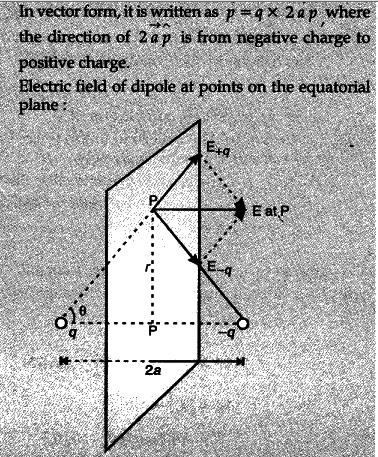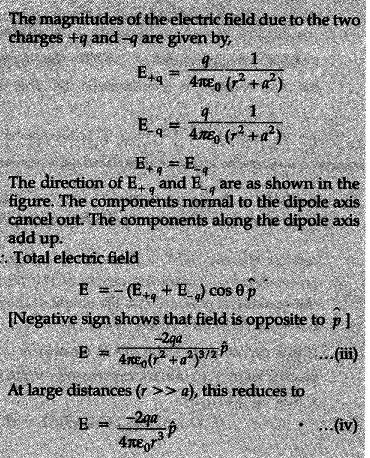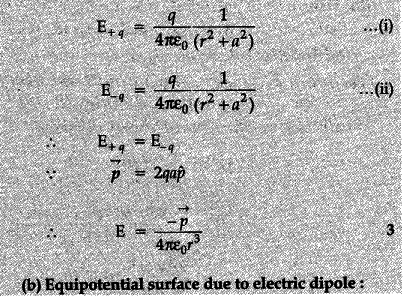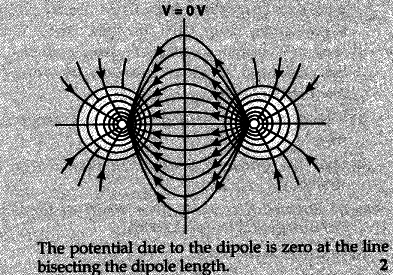# Define electric dipole moment. Is it a scalar or a vector?

Define electric dipole moment. Is it a scalar or a vector ? Derive the expression for the electric field of a dipole at a point on the equatorial plane of the dipole.
(b) Draw the equipotential surfaces due to an electric dipole. Locate the points where the potential due to the dipole is zero.

(a) Electric dipole moment : The strength of an electric dipole is measured by the quantity electric dipole moment. Its magnitude is equal to the product of the magnitude of either charge and the distance between the two charges.